# Practice Questions - Sound, Class 8, Science, | EduRev Notes

## Class 9 : Practice Questions - Sound, Class 8, Science, | EduRev Notes

The document Practice Questions - Sound, Class 8, Science, | EduRev Notes is a part of the Class 9 Course Science Class 9.
All you need of Class 9 at this link: Class 9

Solved Examples
Q1. A source of wave produces 40 crests and 40 troughs in 0.4 second. Find the frequency of the wave.
Sol. Number of crests and troughs produced by the wave = 40
Number of waves formed = 40
Time taken = 0.4 s, Frequency = ?
Number of waves produced in one second = 100 s-1
Frequency of the wave = 100 Hz

Q2. A person has a hearing range from 20 Hz to 20 kHz. What are the typical wavelengths of sound waves in air corresponding to these two frequencies? Take the speed of sound in air as 344 m s-1.
Sol. Hearing range = 20 Hz to 20 kHz (= 20000 Hz)
Speed of sound in the air = 344 ms-1
For a wave,
Wavelength = v/ν

So, for v = 20Hz = 20s-1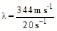=17.2m

and for v = 20000 Hz = 20000 s-1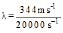=0.0172m = 1.72 cm

Q3. Calculate the wavelength of a sound wave whose frequency is 220 Hz and speed is 440 m/s in a given medium.
Sol. Frequency, v = 220 Hz
Speed of sound, v = 440 m/ s
The wavelength can be described by the relationship,
Wave velocity = Wavelength of the wave x Frequency of the wave

440 m s-1 = λ × 220 Hz = λ × 220 s-1

So λ =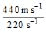= 2 m

Therefore, wavelength of the sound wave is 2 m.

Q4. A person is listening to sound of 50 Hz sitting at a distance of 450 m from the source of sound. What is the time interval between successive compressions from the source reaches him?
Sol. Frequency of the sound = 50 Hz
Distance from the source = 450 m
Time between the successive compressions is equal to time taken by the sound to travel a distance equal to its wavelength. Thus, we have to find out the time period we know,

Time period, T = 1/frequency

So T =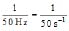= 0.02 s

The successive compressions will reach the person after every 0.02 s.

Q5. A human heart, on an average, is found to beat 75 times a minute. Calculate its frequency.
Sol. No. of beats of human heart = 75 min-1 =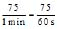= 1.25 s-1
So, Average frequency of human heart beating = 1.25 s-1

Q6. A boat at anchor is rocked by waves whose consecutive crests are 100 m apart. The wave velocity of the moving crests is 20 m/ s. What is the frequency of rocking of the boat?
Sol. Distance between two consecutive crests = 100 m

Wave velocity v = 20 m/ s
The distance between two consecutive crests is equal to the wavelength of the

wave.
So, Frequency =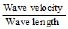=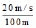= 0.2 s-1

So, the frequency of rocking of the boat is 0.2 s-1.

Q7. A longitudinal wave is produced on a toy slinky. The wave travels at a speed of 30 cm/ s and the frequency of the wave is 20 Hz. What is the minimum separation between the consecutive compressions of the slinky?
Sol. Wave speed, v = 30 cm/ s
Frequency of the wave, = 20 Hz = 20 s-1
The minimum separation between the consecutive compressions is equal to the wavelength.
Therefore,Wavelength =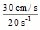= 1.5 cm

Thus, the minimum separation between the consecutive compression of the slinky is 1.5 cm.

Q8. A bat can hear sound at frequencies up to 120 kHz. Determine the wavelength of sound in the air at this frequency. Take the speed of sound in the air as 344 m/s.
Sol. Frequency, v = 120 kHz = 120 × 103 Hz = 120 × 103 s-1
Velocity of sound in the air, v = 344 m/s
Wavelength of the sound wave, λ = ?

We know,

Wavelength, λ =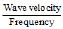=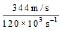= 2.87 × 10-3 m = 0.29 cm

Q9. A gun is fired in the air at a distance of 660 m, from a person. He hears the sound of the gun after 2 s. What is the speed of sound?
Sol. Distance travelled by sound = 660 m, Time taken by the sound = 2 s, Speed of sound in air = ?

So, Speed of sound =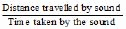Speed of sound =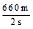= 330 m/s

Thus, the speed of sound in the air is 330 m/s.

Q10. A child hears an echo from a cliff 4 seconds after the sound from a powerful cracker is produced. How far away is the cliff from the child? Velocity of sound in air at 20°C is 344 m/ s.
Sol. Let the distance between the child and the cliff be d. Then,
Total distance travelled by the sound = 2d
Total time taken by the sound = 4s
Then, Velocity of sound = 344m/s
This gives, d = 344 m/s × 2s = 688 m

Thus, the cliff is at a distance of 688 m from the child.

Q11. A ship sends on a high frequency sound wave and receives an echo after 1 second. What is the depth of the sea? Speed of sound in water is 1500 m/s.
Sol. Let,
Depth of the sea = d
So, Total distance travelled by the sound wave = 2d
Time taken by sound to travel both ways = 1s
As per definition
Speed of the sound = 1500m/s
Then, 1500 m/s =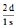or d =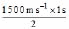= 750 m

Thus the depth of the sea is 750 metres.

Q12. A sonar echo takes 2.2 s to return from a whale. How far away is the whale?
Sol. Total time taken by the signal = 2.2 s
So, Time taken the signal to reach the whale = = 1.1 s
Distance of the whale = d
From the literature, speed of sound in sea water at 25°C = 1533 ms-1
So, Distance of the whale, d = Speed of the signal x Time taken
or d = 1533 m s-1 × 1.1 s = 1686.3 m

Q13. Using the SONAR, sound pulses are emitted at the surface of water. These pulses after being reflected from the bottom are detected. If the time interval from the emission to the detection of the sound pulses is 2 seconds, find the depth of the water. Velocity of sound in water = 1498 m/s.
Sol. Let, depth of the water from the earth's surface be d. Then,
Total distance travelled by the pulse = 2d
Total time taken by the pulse = 2 s
As per definition,
So, Velocity of the sound =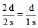Velocity =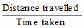1498 m/s =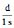This gives,
d = 1498 m/s × 1s = 1498 m
Thus, the depth of water is 1498 m.

Q14. A wave moves a distance of 8 m in 0.05 s.
(a) Find the velocity of the wave.
(b) What is the wavelength of the wave if its frequency is 200 Hz ?
Sol. (a) Velocity = =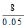= 160 ms-1
(b) λ = v/v = 0.8m

Q15. Two children are at opposite ends of an iron pipe. One strikes his end of the iron pipe with a stone. Find the ratio of times taken by the sound waves in air and in iron to reach the other child. Given velocity of sound in air is 344 ms-1 and that in iron is 5130 ms-1.
Sol. Here, the distance travelled by sound waves through air and iron is same which is equal to the thickness (s) of the rod.

For air, jet the speed be v1 and time be t1.

For iron, let the speed be v2 and time be t2.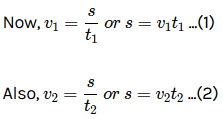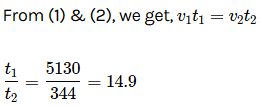Q16. A boat at anchor is rocked by waves whose consecutive crests are 100 m apart. The wave velocity of the moving crests is 20 m/s. What is the frequency of rocking of the boat?
Sol. Given λ = 100 m, n = 20 m/s
Now v = n/λ

v =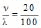= 0.2 Hz

Q17. A stone is dropped into a well 44.1 m deep. The splash is heard 3.13 seconds after the stone is dropped. Find the velocity of sound in air.
Sol. For downward motion of the stone: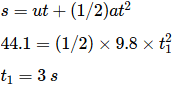For reflected sound wave: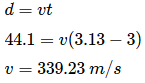Q18. Using sonar, sound pulses are emitted at the surface of water. These pulses after being reflected from water bottom are detected. If the time interval from the emission to the detection of the sound pulses is 2 seconds, find the depth of the water. [speed of sound in water = 1531 m/s given].
Sol. Distance traveled by the sound between emission and detection will be two times of depth (d).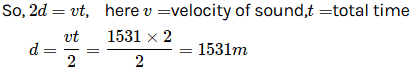Offer running on EduRev: Apply code STAYHOME200 to get INR 200 off on our premium plan EduRev Infinity!

## Science Class 9

205 videos|287 docs|135 tests

,

,

,

,

,

,

,

,

,

,

,

,

,

,

,

,

,

,

,

,

,

,

,

,

,

,

,

,

,

,

;